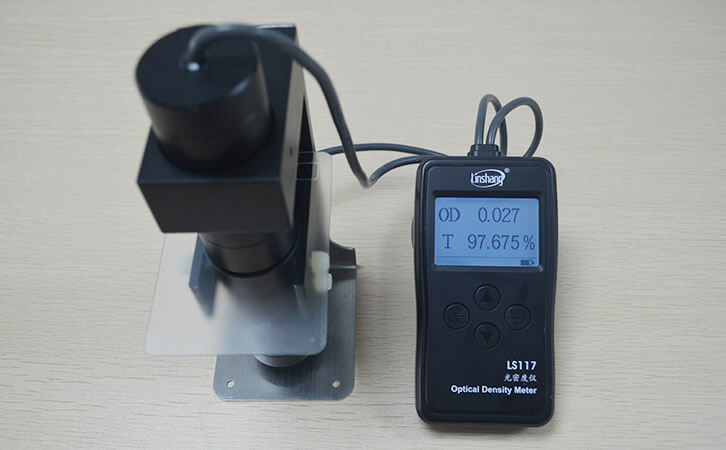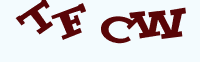# What is the Difference between the Densitometer and the Haze Meter?

Time:2019/11/05 15:08:00 Browse:655

Customers who purchase our densitometers sometimes can't tell the difference between a diffuse transmission densitometer and a haze meter. We often confuse these two different concepts together, so let's take a closer look at the difference between the two meters.

## 1.What is the haze?

First,let's take a look at what is haze.It is the percentage of transmitted light that is above the angle of 2.5°from the incident light as a percentage of the total transmitted light. Usually, only the scattered light flux that is more than 2.5 degrees from the incident light intensity direction is used to calculate the haze.The greater the haze,the lower the gloss and transparency of the material. So the image formation will be affected a lot.

## 2.Working principle of the haze meter

Due to the optical properties and inhomogeneities of the material surface,surface defects, internal tissue inhomogeneities,the presence of bubbles and impurities, etc.The beam changes direction or scatters or deflects as it enters the medium.The part of the chaotic light that we produced is called scattering.Internationally,the ratio of the scattered light flux to the transmitted light flux that deviates from the incident light direction through the sample is expressed as a percentage,which is called haze.

## 3.LS117 densitometer of diffuse transmission principle

First,let's take a look at what is optical density.It is a complex property of a material that slows the speed of light propagation and produces a refractive effect.The optical density value is a logarithmic value, which is the ratio logarithm of the incident light to the transmitted light or the reciprocal logarithm of the light transmittance. Its essence is absorbance.The LS117 densitometer uses the diffuse transmission principle.The probe has an integrating sphere effect,which is the diffuse transmission of light through the rough surface.The transmitted light will spread out in all directions and evenly distributed throughout the hemisphere space.At this time, the brightness is the same in all directions.The optical density value is then calculated by calculating the formula OD(optical density) = log10(incident light/transmitted light) or OD=log10(1/transmittance).
Therefore,the densitometer and the haze meter are fundamentally different.We believe that through the above introduction.You may be more aware of whether you need a densitometer or a haze meter.

Enquiry

### EnquiryClick image refresh captcha
x